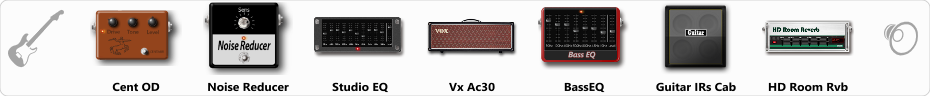# Balanced overdrive[Vox]

Discussion in 'ToneLib-GFX presets' started by Jean C.Guion, Sep 23, 2019.

1. Balanced overdrive[Vox]

Preset name: Balanced overdrive[Vox]

nicely tuned chugy overdrive...lots of bounce with a saturated ,but balanced top end...very feely...{adjust amp drive using 'Studio EQ' output volume} [keeps the bass level clean]...

Effects chain:Effect: "Cent OD" (Overdrive / Distortion), active - "yes"
"Drive" = 24
"Tone" = 81
"Level" = 19

Effect: "Noise Reducer" (Dynamics / Filter), active - "yes"
"Sens" = 30
"Mode" = Hard

Effect: "Studio EQ" (Dynamics / Filter), active - "yes"
"31 Hz" = -3
"62 Hz" = -4
"125 Hz" = -4
"250 Hz" = 2
"500 Hz" = -2
"1 kHz" = -1
"2 kHz" = 2
"4 kHz" = -10
"8 kHz" = -15
"16 kHz" = -15
"above 16 kHz" = 0
"Level (dB)" = 3

Effect: "Vx Ac30" (Amp simulators), active - "yes"
"Gain" = 71
"Bass" = 54
"Middle" = 47
"Treble" = 65
"Presence" = 78
"Master" = 78
"Output" = 50
"Level (dB)" = -10

Effect: "BassEQ" (Dynamics / Filter), active - "yes"
"50 Hz" = 2
"120 Hz" = 0
"400 Hz" = -2
"500 Hz" = -3
"800 Hz" = -2
"4.5 kHz" = -2
"10 kHz" = -3
"Level (dB)" = 7

Effect: "Guitar IRs Cab" (Cabinets), active - "yes"
"Model" = Fender Twin Rev (2x12")
"Mic Position" = Middle
"Mic Distance" = Near
"Low Cut (Hz)" = 56
"Hi Cut (kHz)" = 14.5
"Mix" = 67
"Level (dB)" = 7

Effect: "HD Room Rvb" (Reverb), active - "yes"
"Time" = 6.6
"PreLPF" = 77
"PreDelay" = 60
"HiDamp" = 65
"LoGain" = -1.0
"Mix" = 41

Note: You will need to download and install the ToneLib-GFX software to use the preset.

File size:
830 bytes
Views:
3,686